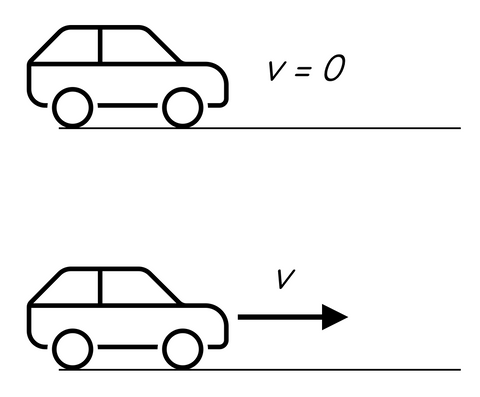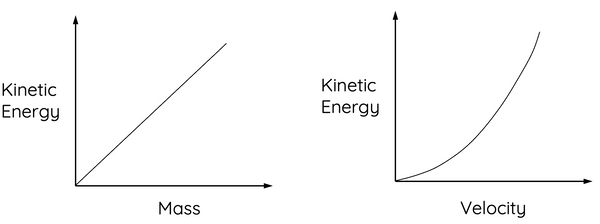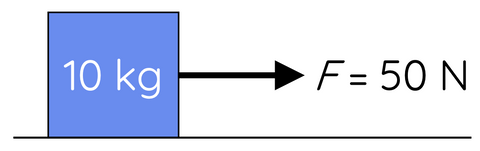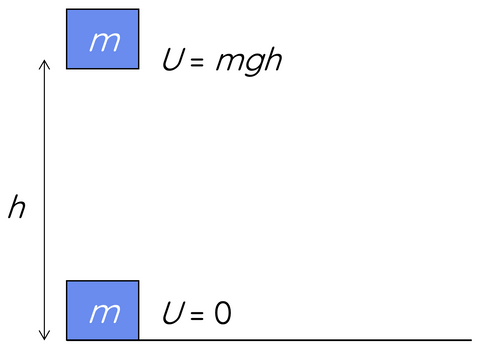# Energy and Work

This topic is part of the HSC Physics course under the section Forces, Acceleration and Energy.

### HSC Physics Syllabus

• apply the special case of conservation of mechanical energy to the quantitative analysis of motion involving:

– work done and change in the kinetic energy of an object undergoing accelerated rectilinear motion in one dimension 𝑊=𝐹𝑠=𝐹𝑠cos𝜃
– changes in gravitational potential energy of an object in a uniform field ∆U = mg∆h

### What is Energy and Work?

This video introduces the concept of energy and work by discussing kinetic energy, gravitational potential energy and mechanical energy.

When studying physics, three fundamental concepts play a crucial role in understanding the world around us: energy, and work. For Year 11 HSC Physics students, mastering these principles is essential to excel in the subject.

### Understanding Work

In physics, work refers to the amount of energy transferred by a force acting on an object over a displacement (s). It is calculated using the following formula:

$$W = Fs \cos{\theta}$$

where:

• W is the work done (measured in Joules, J)
• F is the force applied (measured in Newtons, N)
• is the displacement moved (measured in meters, m)
• θ is the angle between the force and the direction of motion

Work is a scalar quantity. It's important to note that while work is a scalar, the force and displacement involved in the calculation of work are vector quantities, which have both magnitude and direction.

### Calculating Work Done – Example 1Suppose you push a 10 kg box along the floor with a force of 20 N for a distance of 5 meters. The force is exerted horizontally, which means it's in the same direction as the motion. In this case, the angle (θ) between the force and the direction of motion is 0 degrees. Using the formula for work, we can calculate the work done:

$$W = Fs \cos{\theta}$$

$$W = 20 \times 5 \times \cos{0°}$$

$$W = 100 \hspace{2mm} J$$

The work done by you in pushing the box is 100 Joules.

### Calculating Work Done – Example 2Suppose you push a 10 kg box along the floor with a force of 20 N at an angle of 30º above the horizontal for a distance of 5 meters. Using the formula for work, we can calculate the work done:

$$W = Fs \cos{\theta}$$

$$W = 20 \times 5 \times \cos{30°}$$

$$W = 87 \hspace{2mm} J$$

The work done by you in pushing the box is 87 Joules.

When the same magnitude of force is applied at an angle i.e., not parallel to the displacement of the mass, the work done is smaller.

### Grasping the Concept of Energy

Energy is a fundamental property that represents the capacity of an object or system to bring about changes in itself or its surroundings. In physics, energy is often described as the ability to perform work or to cause motion. Energy is a scalar quantity and is always conserved, meaning that it can be transferred or converted from one form to another but cannot be created or destroyed.

The SI unit of energy is the Joule (J), which is also the unit of work.

It is important to remember that energy can manifest itself in various ways, which is why there are different forms of energy. However, regardless of the form, energy always represents the capacity to bring about changes or to perform work.

Mechanical energy forms includes:

• Kinetic energy
• Potential energy (there are also different types of potential energy)

Non-mechanical energy forms includes

• Chemical energy
• Thermal energy (heat)
• Acoustic energy (sound)
• Electrical energy
• Nuclear energy

### Kinetic Energy

Kinetic energy is the energy possessed by an object due to its motion. The amount of kinetic energy an object has depends on its mass and velocity. The formula for calculating kinetic energy is:

$$K = \frac{1}{2}mv^2$$

where:

• K is the kinetic energy (measured in Joules, J)
• m is the mass of the object (measured in kilograms, kg)
• v is the velocity of the object (measured in meters per second, m/s)Kinetic energy is directly proportional to mass, and velocity squared. Graphically, this implies the relationship between kinetic energy and mass is linear and velocity is parabolic as shown below.The faster an object moves or the more massive it is, the greater its kinetic energy will be. When an object comes to a stop or is at rest, its kinetic energy is zero.

### Calculating Kinetic Energy

What is the kinetic energy of a 5 kg mass moving at a constant speed of 2 m/s?

$$K = \frac{1}{2}mv^2$$

$$K = \frac{1}{2}(5)(2)^2$$

$$K = 10 \hspace{2mm} J$$

### Work and Kinetic Energy – Example

When work is done on a mass to cause motion, energy is transferred to its kinetic energy. Thus, work equals the change in the mass's kinetic energy.Suppose you push a 10 kg box, initially at rest, along the floor with a force of 50 N horizontally for a distance of 4 metres. What is the final kinetic energy of the mass and its final velocity?

$$W = Fs \cos{\theta}$$

$$W = 50 \times 4 \times \cos{0°}$$

$$W = 200 \hspace{2mm} J$$

The work done is also given by:

$$W = \Delta K = \frac{1}{2}mv^2 - \frac{1}{2}mu^2$$

$$200 = \frac{1}{2} \times 10 \times v^2 - \frac{1}{2} \times 10 \times 0^2$$

$$v = 6.3 \hspace{2mm} m \hspace{1mm} s^{-1}$$

Therefore, the final kinetic energy of the box is 200 J, and the final velocity is 6.3 m/s.

### Gravitational Potential Energy

Gravitational potential energy is the energy an object possesses due to its position in a gravitational field, such as its height above the ground.The formula for calculating gravitational potential energy is:

$$\Delta U = mg \Delta h$$

where:

• ∆U is the change in potential energy (measured in Joules, J)
• m is the mass of the object (measured in kilograms, kg)
• g is the acceleration due to gravity (approximately 9.8 m/s²)
• ∆h is the height of the object above a reference point (measured in meters, m)

An object's gravitational potential energy increases as it is raised higher above the ground and decreases as it descends.

The change in gravitational potential energy (∆U) when a mass moves closer to the surface of Earth can also be described as the work done by gravity.

### Calculating Gravitational Potential Energy

(a) What is the gravitational potential energy of a 3 kg mass 15 m above the surface of Earth?

$$U = mgh$$

$$U = (3)(9.8)(15)$$

$$U = 441 \hspace{2mm} J$$

(b) What is the change in its gravitational potential energy when it falls to a height of 5 m?

$$\Delta U = mg \Delta h$$

$$\Delta U = (3)(9.8)(15 - 5)$$

$$\Delta U = 294 \hspace{2mm} J$$

Therefore, gravity does 294 J of work on the mass as it moves from 15 m to 5 m above the surface of Earth.